# Helical calculus

(diff) ← Older revision | Latest revision (diff) | Newer revision → (diff)

screw calculus

A branch of vector calculus dealing with operations on screws, which are ordered pairs of collinear vectorshaving their origins at a common point. The vectoris called the screw vector, the axis defined by this vector is called the screw axis,is the screw moment, while the numberin the equation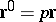is said to be the screw parameter.

Helical calculus deals with the operations of addition of screws, multiplication by a number, scalar and vector products, etc. In this context, the operations of helical calculus are reduced to operations on complex vectors of the form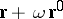where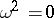; the complex number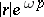is said to be the complex modulus of the screw; the number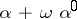is said to be the complex angle between the screws (is the angle between the axes, whileis the distance between them). All formulas of helical calculus are identical with the formulas of vector calculus if the modulus of the vector is replaced by the complex modulus of the screw, and the ordinary angle between straight lines is replaced by the complex angle.

For instance, the scalar product of two screws is equal to the product of their complex moduli and the cosine of the complex angle between them (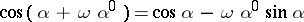); the screw product of two screws is a screw whose axis is perpendicular to the axes of the factors; a vector has the direction of the vector product of its factors, while the complex modulus is equal to the product of the complex moduli of the screws and the sine of the complex angle between the axes of the factors (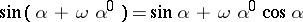). The correspondence between the formulas of vector analysis and those of helical calculus, which involve complex scalar functions and screw functions of screw arguments, is established in a similar manner.

Helical calculus is employed in mechanics, where arbitrary displacements of a solid body or an arbitrary system of forces acting on a body may be expressed by screws , and in geometry in the theory of ruled surfaces , .

Helical calculus was born in the early 19th century following the studies of L. Poinsot, M. Chasles, A. Möbius, and J. Plücker; the first major treatise was due to R. Ball . Helical calculus proper was developed by A.P. Kotel'nikov .

How to Cite This Entry:
Helical calculus. Encyclopedia of Mathematics. URL: http://encyclopediaofmath.org/index.php?title=Helical_calculus&oldid=17388
This article was adapted from an original article by A.B. Ivanov (originator), which appeared in Encyclopedia of Mathematics - ISBN 1402006098. See original article